Courses

RD Sharma Solutions -Ex-17.2, Constructions, Class 9, Maths Notes | Study RD Sharma Solutions for Class 9 Mathematics - Class 9

Class 9: RD Sharma Solutions -Ex-17.2, Constructions, Class 9, Maths Notes | Study RD Sharma Solutions for Class 9 Mathematics - Class 9

The document RD Sharma Solutions -Ex-17.2, Constructions, Class 9, Maths Notes | Study RD Sharma Solutions for Class 9 Mathematics - Class 9 is a part of the Class 9 Course RD Sharma Solutions for Class 9 Mathematics.
All you need of Class 9 at this link: Class 9

Q1. Draw an angle and label it as ∠BAC. Construct another angle, equal to ∠BAC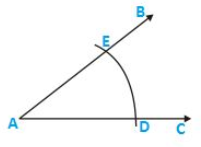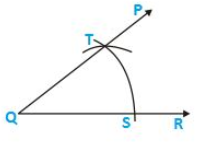Steps of construction:

1. Draw an angle ABC and a line segment QR.
2. With center A and any radius, draw an arc which intersects ∠BACat E and D.
3. With Q as a centre and same radius draw an arc which intersects QR at S.
4. With S as center and radius equal to DE, draw an arc which intersects the previous arc at T.
5. Draw a line segment joining Q and T.

Therefore ∠PQR= ∠BAC

Q2. Draw an obtuse angle. Bisect it. Measure each of the angles so formed.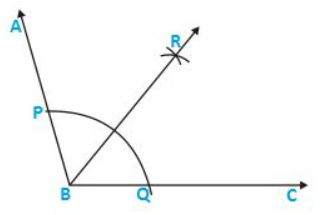Steps of construction:

1. Draw an angle ∠ABC of 1200.
2. With B as a centre and any radius, draw an arc which intersects AB at P and BC at Q.
3. With P as center and radius more than half of PQ draw an arc.
4. With Q as a center and same radius draw an arc which cuts the previous arc at R.
5. Join BR.

Therefore ∠ABR=  ∠RBC=  600

Q3. Using your protractor, draw an angle of 1080. With this given angle as given, draw an angle of 540.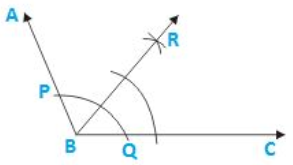Steps of construction:

1. Draw an angle ABC of 1080.
2. With B as the center and any radius draw an arc which intersects AB at P and BC at Q.
3. With P as center and radius more than half of PQ draw an arc.
4. With Q as the centre and same radius draw an arc which intersects the previous arc at R.
5. Join BR.

Therefore ∠RBC=  540

Q4. Using the protractor, draw a right angle. Bisect it to get an angle of measure 450.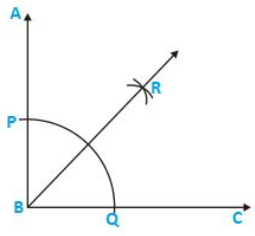Steps of construction:

1. Draw an angle ABC of 900.
2. With B as the centre and any radius draw an arc which intersects AB at P and BC at Q.
3. With P as center and radius more than half of PQ draw an arc.
4. With Q as center and same radius draw an arc which intersects the previous arc at R.
5. Join RB.

Therefore ∠RBC=  450

Q5. Draw a linear pair of angles. Bisect each of the two angles. Verify that the two bisecting rays are perpendicular to each other.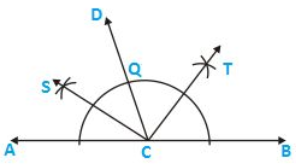Steps of construction:

1. Draw two angles DCA and DCB forming linear pair
2. With center C and any radius draw an arc which intersects AC at P and CD at Q and CB at R
3. With center P and Q and any radius draw two arcs which intersect each other at S
4. Join SC
5. With Q and R as center and any radius draw two arcs which intersect each other at T
6. Join TC

Therefore ∠SCT=  900.

Q6. Draw a pair of vertically opposite angles. Bisect each of the two angles. Verify that the bisecting rays are in the same line.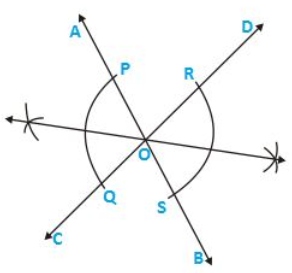Steps of Construction:

1. Draw a pair of vertically opposite angle ∠AOC and ∠DOB.
2. Keeping O as the center and any radius draw two arcs which intersect OA at P, OC at Q, OB at S and OD at R.
3. Keeping P and Q as center and radius more than half of PQ draw two arcs which intersect each other at T.
4. Join TO.
5. Keeping R and S as center and radius more than half of RS draw two arcs which intersect each other at U.
6. Join OU.

Therefore TOU is a straight line

Q7. Using rulers and compasses only, draw a right angle.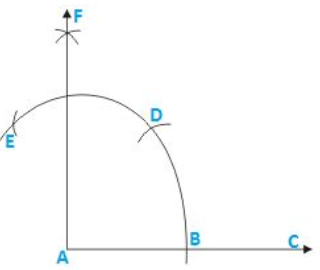Steps of construction:

1. Draw a line segment AB.
2. Keeping A as the center and any radius draw an arc which intersects AB at C.
3. Keeping C as center and the same radius draw an arc which intersects the previous arc at D.
4. Keeping D as the center and same radius draw an arc which intersects arc in (2) at E.
5. Keeping E and D as center and radius more than half of ED draw arcs which intersect each other at F.
6. Join FA.

Therefore ∠FAB=  900

Q8.Using rulers and compasses only, draw an angle of measure 1350.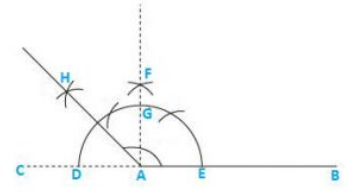Steps of construction:

1. Draw a line segment AB and produce BA to C.
2. Keeping A as the center and any radius draw an arc which intersects AC at D and AB at E.
3. Keeping D and E as center and radius more than half of DE draw arcs which intersect each other at F.
4. Join FA which intersects the arc in (2) at G.
5. Keeping G and D as center and radius more than half of GD draw arcs which intersect each other at H.
6. Join HA.

Therefore ∠HAB=  1350

Q9. Using a protractor, draw an angle of measure 720. With this angle as given draw angles of measure 360and 540.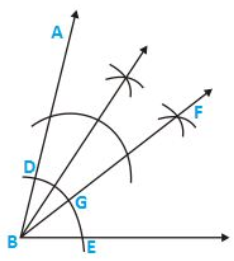Steps of construction:

1. Draw an ∠ABC of 720 with the help of a protractor.
2. Keeping B as center and any radius draw an arc which intersects AB at D and BC at E.
3. Keeping D and E as center and radius more than half of DE draw two arcs which intersect each other at F.
4. Join FB which intersects the arc in (2) at G.
5. Keeping D and G as center and radius more than half of DG draw two arcs which intersect each other at H
6. Join HB

Therefore ∠HBC=  540

∠FBC=  360

Q10. Construct the following angles at the initial point of a given ray and justify the construction:

1. 450
2. 900

1. 450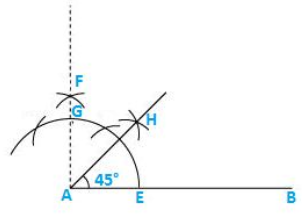Steps of construction:

1. Draw a line segment AB and produce BA to C.
2. Keeping A as the center and any radius draw an arc which intersects AC at D and AB at E.
3. Keeping D and E as center and radius more than half of DE draw arcs which intersect each other at F.
4. Join FA which intersects the arc in (2) at G.
5. Keeping G and E as center and radius more than half of GE draw arcs which intersect each other at H.
6. Join HA.

Therefore ∠HAB=  450

2. 900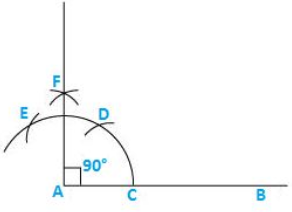Steps of construction

1. Draw a line segment AB.
2. Keeping A as the center and any radius draw an arc which intersects AB at C.
3. Keeping C as center and the same radius draw an arc which intersects the previous arc at D.
4. Keeping D as the center and same radius draw an arc which intersects arc in (2) at E.
5. Keeping E and D as center and radius more than half of ED draw arcs which intersect each other at F.
6. Join FA.

Therefore ∠FAB=  900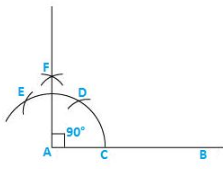Q11. Construct the angles of the following measurements:

1. 300
2. 750
3. 1050
4. 1350
5. 150
6.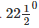1. 300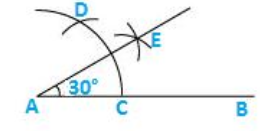Steps of construction:

1. Draw a line segment AB.
2. Keeping A as the centre and any radius draw an arc which intersects AB at C.
3. Keeping C as center and the same radius draw an arc which intersects the previous arc at D.
4. Keeping D and C as center and radius more than half of DC draw arcs which intersect each other at E.
5. Join EA.

Therefore ∠EAB=  300

2. 750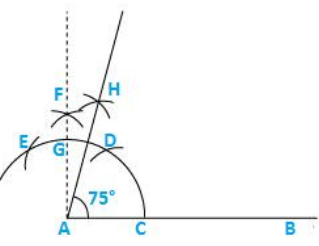Steps of construction:

1. Draw a line segment AB
2. Keeping A as center and any radius draw an arc which intersects AB at C
3. Keeping C as center and the same radius draw an arc which intersects the previous arc at D
4. Keeping D as center and same radius draw an arc which intersects arc in (2) at E
5. Keeping E and D as center and radius more than half of ED, draw arcs intersecting each other at F
6. Join FA which intersects arc in (2) at G
7. Keeping G and D as center and radius more than half of GD draw arcs intersecting each other at H
8. Join HA

Therefore ∠HAB=  750

3.1050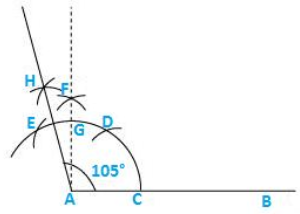Steps of construction:

1. Draw a line segment AB.
2. Keeping A as the center and any radius draw an arc which intersects AB at C.
3. Keeping C as center and the same radius draw an arc which intersects the previous arc at D.
4. Keeping D as the centre and same radius draw an arc which intersects arc in (2) at E.
5. Keeping E and D as center and radius more than half of ED draw arcs which intersect each other at F.
6. Join FA which intersects arc in (2) at G.
7. Keeping E and G as center and radius more than half of EG draw arcs which intersect each other at H.
8. Join HA.

Therefore ∠HAB=  1050

4. 1350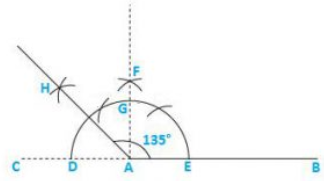Steps of construction:

1. Draw a line segment AB and produce BA to C.
2. Keeping A as the center and any radius draw an arc which intersects AC at D and AB at E.
3. Keeping D and E as center and radius more than half of DE draw arcs which intersect each other at F.
4. Join FA which intersects the arc in (2) at G.
5. Keeping G and D as center and radius more than half of GD draw arcs which intersect each other at H
6. Join HA.

Therefore ∠HAB=  1350

5. 150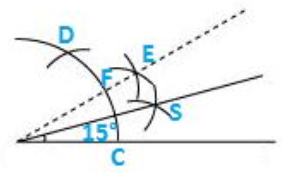Steps of construction:

1. Draw a line segment AB.
2. Keeping A as the centre and any radius draw an arc which intersects AB at C.
3. Keeping C as center and the same radius draw an arc which intersects the previous arc at D.
4. Keeping D and C as center and radius more than half of DC draw arcs which intersect each other at E.
5. Join EA which intersects arc in (2) at F.
6. Keeping F and C as center and radius more than half of FC draw arcs which intersect each other at G.
7. Join GA.

Therefore ∠GAB=  150

6.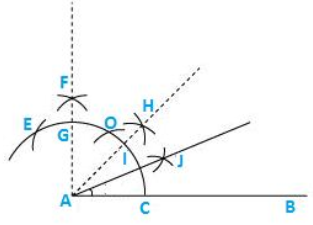Steps of construction:

1. Draw a line segment AB.
2. Keeping A as the center and any radius draw an arc which intersects AB at C.
3. Keeping C as center and the same radius draw an arc which intersects the previous arc at D.
4. Keeping D as the centre and same radius draw an arc which intersects arc in (2) at E.
5. Keeping E and D as center and radius more than half of ED draw arcs which intersect each at F.
6. Join FA which intersects arc in (2) at G.
7. Keeping G and C as center and radius more than half of GC draw arcs intersecting each other at point H.
8. Join HA which intersects the arc in (2) at a point I.
9. Keeping I and C as center and radius more than half of IC draw arcs intersecting each other at point J.
10. Join JA.

Therefore ∠JAB=The document RD Sharma Solutions -Ex-17.2, Constructions, Class 9, Maths Notes | Study RD Sharma Solutions for Class 9 Mathematics - Class 9 is a part of the Class 9 Course RD Sharma Solutions for Class 9 Mathematics.
All you need of Class 9 at this link: Class 9Use Code STAYHOME200 and get INR 200 additional OFF Use Coupon Code

Top Courses for Class 9RD Sharma Solutions for Class 9 Mathematics

91 docs

Top Courses for Class 9Track your progress, build streaks, highlight & save important lessons and more!

,

,

,

,

,

,

,

,

,

,

,

,

,

,

,

,

,

,

,

,

,

,

,

,

,

,

,

,

,

,

;